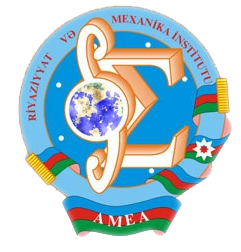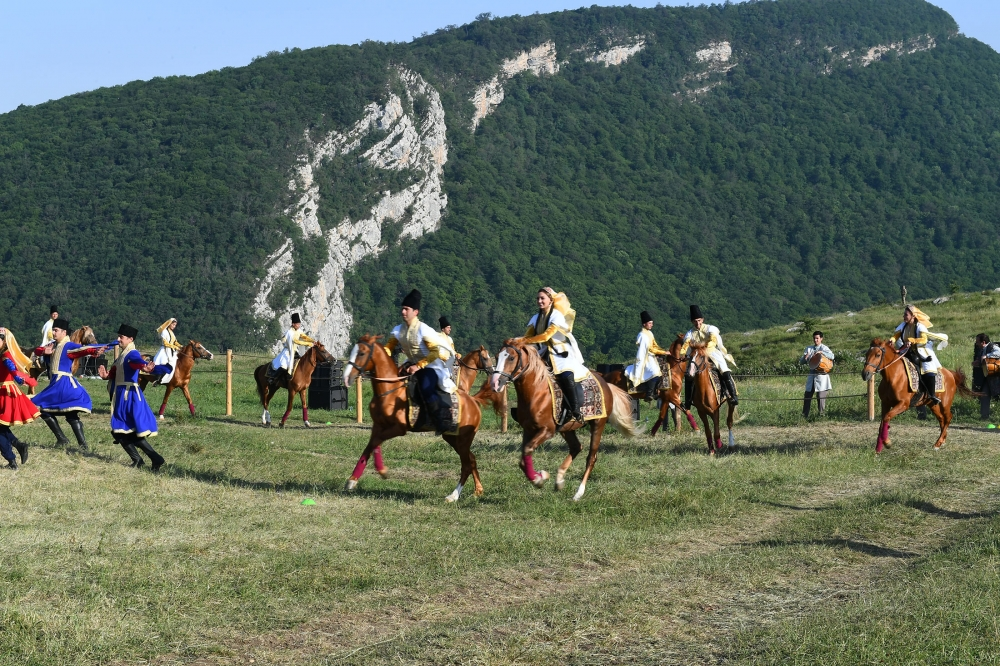Riyaziyyat və Mexanika İnstitutu

## Qarabağ xəbərləri### Şuşada Heydər Əliyev Fondunun təşkilatçılığı ilə “Musiqi irsi və Qarabağ atları Cıdır düzündə” adlı kompozisiya təqdim olunub## Proceedings 2003/19/XXVII

 Year: 2003 Volume: XIX Number: XXVII MATHEMATICS Ahmedov A.M., Bashar F. Abstract [PDF] On spectrum of the Cesaro operator [PDF] 3 Aliyev S.A. Abstract [PDF] Convergence of multitype continuous time branching processes [PDF] 9 Amanov R.A. Abstract [PDF] Estimation of Green function of the first boundary value problem for the second order nonuniformly degenerated divergent elliptic equations [PDF] 15 Aral A. Abstract [PDF] Approximation by Ibragimov-Gadjiev operators in polynomial weighted space [PDF] 35 Babayev M-B.A., Novruzova Is.A. Abstract [PDF] On de la Valle-Poussin type theorem [PDF] 45 Bandaliyev R.A. Abstract [PDF] Two-weighted inequalities of weak type for some anisotropic integral operator on the domains in R [PDF] 49 E.R.Gasimova Abstract [PDF] Coercive estimate for degenerated elliptic-parabolic operators of the second order [PDF] 59 Hajiyev A.H., Ibadova I.A. Abstract [PDF] Mathematical models ofqueueing systems with cyclic services [PDF] 75 Hasanova S.H. Abstract [PDF] The first boundary value problem for Cordes type linear non-vergent parabolic equations of the second order [PDF] 81 Hassanpour A.S. Abstract [PDF] On uniqueness of strong solution of Dirichlet problem for second order quasilinear elliptic equations [PDF] 101 Ismailov A.I. Abstract [PDF] Investigations of classical solution im kleinen of inverse boundary-value problem for semilinear hyperbolic equations of the second order [PDF] 107 Ismailov V.E. Abstract [PDF] On behaviour of the best approximation as a function of an approximation set[PDF] 113 Mamedov I.T., Guliyev D.M. Abstract [PDF] Removable sets of solutions of the second order degenerate elliptic equations of divergence structure [PDF] 117 Mamedov I.T., Mirheydarli M.M. Abstract [PDF] Neumann problem for second order nondivergent elliptic equations with discontinuous coefficients [PDF] 135 Mamedov I.T., Mushtagov F.M. Abstract [PDF] New proof of Garofalo-Lanconelli regularity criterion for the second order parabolic equations of divergent structure II [PDF] 153 Mamedli K.A. Abstract [PDF] On solvability of a mixed problem for a hyperbolic system when the corresponding spectral problent is almost regular [PDF] 171 Nabiev I.M. Abstract [PDF] The inverse periodic problem for the Dirac operator [PDF] 177 Najafov A.M. Abstract [PDF] Interpolation theorem, for LizorHn- Triebel- Morrey type spaces witli dominant mixed derivatives [PDF] 181 Pashayeva E.E. Abstract [PDF] Multiple expansion on the whole axis for one family of not self-adjoint singular differential operators of complicated form [PDF] 187 Salmanov V.F. Abstract [PDF] On Holder property of solution of some Riemann problem with discontinuous coefficient [PDF] 193 Veliyev S.H. Abstract [PDF] Completeness of some “degenerating” exponent systems in Lp (-7r,7r), (1< p < oo) [PDF] 199 MECHANICS Abbasov A.N. Abstract [PDF] Investigation of two-phase Alteration problem of noncomprcssible viscous fluids in porous medium [PDF] 205 Agalarova N.D. Abstract [PDF] Motion of fluid with free surface under tlie action of cylindrical body[PDF] 213 Alimamedov R.G. Abstract [PDF] Delayed fracture by tensioning inherently elastic thick plate with two-sided exterior deep recess [PDF] 217 Babanly V.Yu. Abstract [PDF] On motion of one phase of three-phase system in porous medium with two obstructions [PDF] 225 Fatuilaeva L.F. Abstract [PDF] On one approximate method of solution of buckling problem nonlinear elastic ring of nonuniform by width [PDF] 233 Gulgazli A.S., Isayev D.I. Abstract [PDF] Canonical form of a yield surface at repeated loading[PDF] 239 Ramazanov T.K., Agayeva S.A. Abstract [PDF] Basic macrononequilibrium equations of multiphase fUtration of multicomponent mixtures in deformable porous media [PDF] 243 APPLIED PROBLEMS OF MATHEMATICS AND MECHANICS Aliyev A.A. Abstract [PDF] Two phase locking protocol in distributed databases [PDF] 251 Huseynov S.A., Mamedzade R.A., Shariati A.Y. Abstract [PDF] Numerical solution of identification problems of heat-exchange process in oil strata [PDF] 257 Kerimov M.Z., Yusubov C.A. Abstract [PDF] Numerical method of analysis of nonstationary processes in asmain pipelines[PDF] 263 Mukhtarov M.A. Abstract [PDF] Solution of stationary axisymetric gravity [PDF] 271 Pirmamedov F.K., Naibova M.K., O.M.Manafly Abstract [PDF] Numerical method of solution of water-oil displacement problem in the presence of several oil- drainage boundaries in bounded space [PDF] 277
Azərbaycanda COVID-19 ilə bağlı statistika
• Virusa yoluxan

596973

• Sağalan

565521

• Yeni yoluxan

0

• Aktiv xəstə

23469

• Ölüm halı

7983

• Test edilib

5,608,158